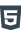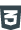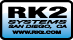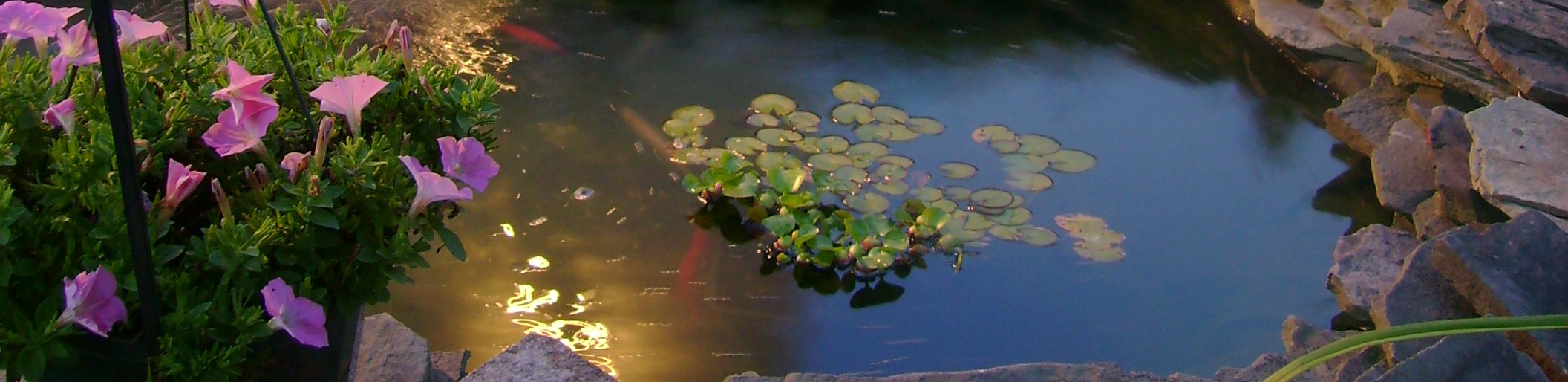Creating Quality Water
Sales
M-F
9AM - 5PM (EST)
760.746.7400
sales@RK2.com
www.RK2Ponds.com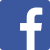# Pond Size

## Calculating the Size of Your Pond

Determines the proper size and style of your pond filtration needs!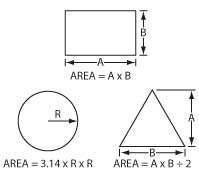### Rectangle Pond

Pond Size Formulas (A feet B feet) = Surface Area ft²
Surface Area ft² x C ft (Average Depth) = Volume ft²
Volume ft³ x 7.5 = Total Pond Gallons

### Circle Pond

(R feet x R feet x 3.14) = Surface Area ft²
Surface Area ft² x B ft (Average Depth) = Volume ft³
Volume ft³ x 7.5 = Total Pond Gallons

### Equilateral Triangle

(A feet x B feet) \$alt246; 2 = Surface Area ft²
Surface Area ft² x B ft (Average Depth) = Volume ft³
Volume ft³ x 7.5 = Total Pond Gallons